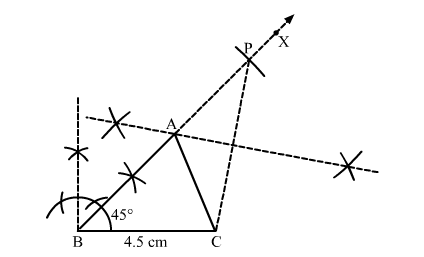# Construct a ∆ABC in which BC = 4.5 cm, ∠B = 45° and AB + AC = 8 cm.`
Question:

Construct a ∆ABC in which BC = 4.5 cm, ∠= 45° and AB AC = 8 cm. Justify your construction.

Solution:Steps of construction:
1. Draw a line segment BC = 4.5 cm.

2. Construct $\angle C B X=45^{\circ}$.

3. Set off BP = 8 cm.
4. Join PC.
5. Draw the right bisector of PC, meeting BP at A.
6. Join AC.

Thus, $\triangle A B C$ is the required triangle.

Justification:

In $\triangle \mathrm{APC}$,

$\angle \mathrm{ACP}=\angle \mathrm{APC}$      (By construction)

⇒ AC = AP                    (Sides opposite to equal angles are equal)
Now
AB = BP − AP = BP − AC
⇒ AB + AC = BP